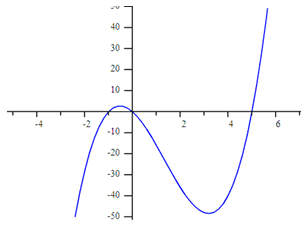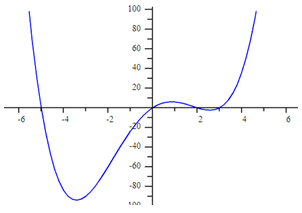Graphs

Graphs

 Site: TBAISD Moodle Course: Michigan Algebra I Preview 2012 Book: Graphs Printed by: Guest user Date: Thursday, November 14, 2019, 01:52 AM

From A Graph

The steps to model a polynomial function from a graph are very similar to the steps used when given a table. First, determine the zeros of the function by looking at the graph. Second, turn each zero into a factor of the function. Finally, determine the factored form of the polynomial.

Example 1 Determine the function that models the graph below:Step 1. Determine the zeros of the function.

(-1, 0), (0, 0), (5, 0)

Step 2. Turn each zero into a factor of the function.

Since x = -1, then (x + 1) is a factor of the polynomial.

Since x = 0, then (x) is a factor of the polynomial.

Since x = 5, then ( x - 5) is a factor of the polynomial.

Example 1 Continued

Step 3. Determine the factored form of the polynomial by using another point from the graph. Substitute the point in for (x, y) and solve for the value of a.

y = a (x + 1)(x)(x - 5); point (4, -40)

-40 = a (4 + 1)(4)(4 - 5)

-40 = a (5)(4)(-1)

-40 = a (-20)

2 = a

y = 2 ( x + 1)( x )( x - 5)

Example 2

Determine the function that models the graph below:Step 1. Determine the zeros of the function.

(-5, 0), (0, 0), (2, 0), (3, 0)

Step 2. Turn each zero into a factor of the function.

Since x = -5, then (x + 5) is a factor of the polynomial.

Since x = 0, then (x) is a factor of the polynomial.

Since x = 2, then (x - 2) is a factor of the polynomial.

Since x = 3, then (x - 3) is a factor of the polynomial.

Step 3. Determine the factored form of the polynomial by using another point from the graph. Substitute the point in for (x, y) and solve for the value of a.

y = a (x + 5)(x)(x - 2)(x - 3); point (-2, -60)

-60 = a (-2 + 5)(-2)(-2 - 2)(-2 - 3)

-60 = a (3)(-2)(-4)(-5)

-60 = a (-120)

.5 = a

y = .5 ( x + 5)( x )( x - 2)( x - 3)

Practice

Polynomials Equations Worksheet